# Sampling

Currently, there are 8 functions associated with the sample verb in the sgsR package:

Algorithm Description Reference
sample_srs() Simple random
sample_systematic() Systematic
sample_strat() Stratified Queinnec, White, & Coops (2021)
sample_nc() Nearest centroid Melville & Stone (2016)
sample_clhs() Conditioned Latin hypercube Minasny & McBratney (2006)
sample_balanced() Balanced sampling Grafström, A. Lisic, J (2018)
sample_ahels() Adapted hypercube evaluation of a legacy sample Malone, Minasny, & Brungard (2019)
sample_existing() Sub-sampling an existing sample

## sample_srs

We have demonstrated a simple example of using the sample_srs() function in vignette("sgsR"). We will demonstrate additional examples below.

raster

The input required for sample_srs() is a raster. This means that sraster and mraster are supported for this function.

#--- perform simple random sampling ---#
sample_srs(raster = sraster, # input sraster
nSamp = 200, # number of desired sample units
plot = TRUE) # plot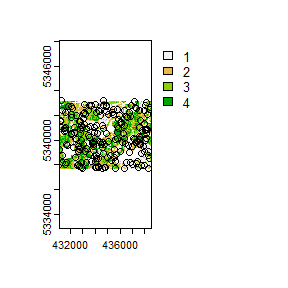#> Simple feature collection with 200 features and 0 fields
#> Geometry type: POINT
#> Dimension:     XY
#> Bounding box:  xmin: 431110 ymin: 5337710 xmax: 438470 ymax: 5343230
#> CRS:           +proj=utm +zone=17 +ellps=GRS80 +units=m +no_defs
#> First 10 features:
#>                  geometry
#> 1  POINT (432070 5340550)
#> 2  POINT (432070 5340550)
#> 3  POINT (434310 5339890)
#> 4  POINT (432270 5339390)
#> 5  POINT (432750 5339250)
#> 6  POINT (438110 5340570)
#> 7  POINT (437650 5339670)
#> 8  POINT (437970 5337950)
#> 9  POINT (431110 5339270)
#> 10 POINT (432930 5337710)
sample_srs(raster = mraster, # input mraster
nSamp = 200, # number of desired sample units
access = access, # define access road network
mindist = 200, # minimum distance sample units must be apart from one another
buff_inner = 50, # inner buffer - no sample units within this distance from road
buff_outer = 200, # outer buffer - no sample units further than this distance from road
plot = TRUE) # plot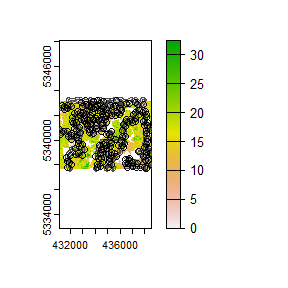#> Simple feature collection with 200 features and 0 fields
#> Geometry type: POINT
#> Dimension:     XY
#> Bounding box:  xmin: 431110 ymin: 5337750 xmax: 438550 ymax: 5343230
#> CRS:           +proj=utm +zone=17 +ellps=GRS80 +units=m +no_defs
#> First 10 features:
#>                  geometry
#> 1  POINT (434850 5339830)
#> 2  POINT (435930 5342050)
#> 3  POINT (437550 5342070)
#> 4  POINT (435070 5337810)
#> 5  POINT (432830 5340650)
#> 6  POINT (433350 5341170)
#> 7  POINT (436010 5342850)
#> 8  POINT (434570 5342090)
#> 9  POINT (433870 5343130)
#> 10 POINT (435650 5342010)

## sample_systematic

The sample_systematic() function applies systematic sampling across an area with the cellsize parameter defining the resolution of the tessellation. The tessellation shape can be modified using the square parameter. Assigning TRUE (default) to the square parameter results in a regular grid and assigning FALSE results in a hexagonal grid.

The location of sample units can also be adjusted using the locations parameter, where centers takes the center, corners takes all corners, and random takes a random location within each tessellation. Random start points and translations are applied when the function is called.

#--- perform grid sampling ---#
sample_systematic(raster = sraster, # input sraster
cellsize = 1000, # grid distance
plot = TRUE) # plot
#--- perform grid sampling ---#
sample_systematic(raster = sraster, # input sraster
cellsize = 500, # grid distance
square = FALSE, # hexagonal tessellation
location = "random", # randomly sample within tessellation
plot = TRUE) # plot
sample_systematic(raster = sraster, # input sraster
cellsize = 500, # grid distance
access = access, # define access road network
buff_outer = 200, # outer buffer - no sample units further than this distance from road
square = FALSE, # hexagonal tessellation
location = "corners", # take corners instead of centers
plot = TRUE)

## sample_strat

The sample_strat() contains two methods to perform sampling:

• "Queinnec" - Hierarchical sampling using a focal window to isolate contiguous groups of stratum pixels, which was originally developed by Martin Queinnec.

• "random" - Traditional stratified random sampling. This method ignores much of the functionality of the algorithm to allow users the capability to use standard stratified random sampling approaches without the use of a focal window to locate contiguous stratum cells.

### method = "Queinnec"

Queinnec, M., White, J. C., & Coops, N. C. (2021). Comparing airborne and spaceborne photon-counting LiDAR canopy structural estimates across different boreal forest types. Remote Sensing of Environment, 262(August 2020), 112510.

This algorithm uses moving window (wrow and wcol parameters) to filter the input sraster to prioritize sample unit allocation to where stratum pixels are spatially grouped, rather than dispersed individuals across the landscape.

Sampling is performed using 2 rules:

• Rule 1 - Sample within spatially grouped stratum pixels. Moving window defined by wrow and wcol.

• Rule 2 - If no additional sample units exist to satisfy desired sample size(nSamp), individual stratum pixels are sampled.

The rule applied to a select each sample unit is defined in the rule attribute of output samples. We give a few examples below:

#--- perform stratified sampling random sampling ---#
sample_strat(sraster = sraster, # input sraster
nSamp = 200, # desired sample size
plot = TRUE) # plot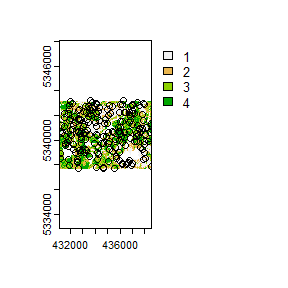#> Simple feature collection with 200 features and 3 fields
#> Geometry type: POINT
#> Dimension:     XY
#> Bounding box:  xmin: 431150 ymin: 5337710 xmax: 438550 ymax: 5343230
#> CRS:           NA
#> First 10 features:
#>    strata type  rule               geometry
#> x       1  new rule1 POINT (436590 5338130)
#> x1      1  new rule2 POINT (436470 5339570)
#> x2      1  new rule2 POINT (438010 5339930)
#> x3      1  new rule2 POINT (437810 5339270)
#> x4      1  new rule2 POINT (437950 5338970)
#> x5      1  new rule2 POINT (434190 5338970)
#> x6      1  new rule2 POINT (436070 5342870)
#> x7      1  new rule2 POINT (433450 5341470)
#> x8      1  new rule2 POINT (433510 5340430)
#> x9      1  new rule2 POINT (434310 5341610)

In some cases, users might want to include an existing sample within the algorithm. In order to adjust the total number of sample units needed per stratum to reflect those already present in existing, we can use the intermediate function extract_strata().

This function uses the sraster and existing sample units and extracts the stratum for each. These sample units can be included within sample_strat(), which adjusts total sample units required per class based on representation in existing.

#--- extract strata values to existing samples ---#
e.sr <- extract_strata(sraster = sraster, # input sraster
existing = existing) # existing samples to add strata value to

TIP!

sample_strat() requires the sraster input to have an attribute named strata and will give an error if it doesn’t.

sample_strat(sraster = sraster, # input sraster
nSamp = 200, # desired sample size
access = access, # define access road network
existing = e.sr, # existing sample with strata values
mindist = 200, # minimum distance sample units must be apart from one another
buff_inner = 50, # inner buffer - no sample units within this distance from road
buff_outer = 200, # outer buffer - no sample units further than this distance from road
plot = TRUE) # plot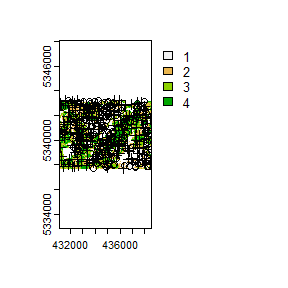#> Simple feature collection with 400 features and 3 fields
#> Geometry type: POINT
#> Dimension:     XY
#> Bounding box:  xmin: 431110 ymin: 5337710 xmax: 438530 ymax: 5343230
#> CRS:           NA
#> First 10 features:
#>    strata     type     rule               geometry
#> 1       1 existing existing POINT (435970 5339490)
#> 2       1 existing existing POINT (436470 5339550)
#> 3       1 existing existing POINT (435550 5342350)
#> 4       1 existing existing POINT (433810 5341570)
#> 5       1 existing existing POINT (436950 5338530)
#> 6       1 existing existing POINT (436670 5338470)
#> 7       1 existing existing POINT (436750 5339070)
#> 8       1 existing existing POINT (436010 5341590)
#> 9       1 existing existing POINT (431970 5341490)
#> 10      1 existing existing POINT (434350 5340890)

The code in the example above defined the mindist parameter, which specifies the minimum euclidean distance that new sample units must be apart from one another.

Notice that the sample units have type and rule attributes which outline whether they are existing or new, and whether rule1 or rule2 were used to select them. If type is existing (a user provided existing sample), rule will be existing as well as seen above.

sample_strat(sraster = sraster, # input
nSamp = 200, # desired sample size
access = access, # define access road network
existing = e.sr, # existing samples with strata values
include = TRUE, # include existing sample in nSamp total
buff_outer = 200, # outer buffer - no samples further than this distance from road
plot = TRUE) # plot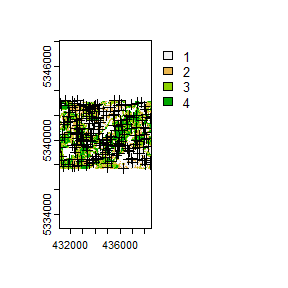#> Simple feature collection with 200 features and 3 fields
#> Geometry type: POINT
#> Dimension:     XY
#> Bounding box:  xmin: 431110 ymin: 5337710 xmax: 438530 ymax: 5343230
#> CRS:           NA
#> First 10 features:
#>    strata     type     rule               geometry
#> 1       1 existing existing POINT (435970 5339490)
#> 2       1 existing existing POINT (436470 5339550)
#> 3       1 existing existing POINT (435550 5342350)
#> 4       1 existing existing POINT (433810 5341570)
#> 5       1 existing existing POINT (436950 5338530)
#> 6       1 existing existing POINT (436670 5338470)
#> 7       1 existing existing POINT (436750 5339070)
#> 8       1 existing existing POINT (436010 5341590)
#> 9       1 existing existing POINT (431970 5341490)
#> 10      1 existing existing POINT (434350 5340890)

The include parameter determines whether existing sample units should be included in the total sample size defined by nSamp. By default, the include parameter is set as FALSE.

### method = "random

Stratified random sampling with equal probability for all cells (using default algorithm values for mindist and no use of access functionality). In essence this method perform the sample_srs algorithm for each stratum separately to meet the specified sample size.

#--- perform stratified sampling random sampling ---#
sample_strat(sraster = sraster, # input sraster
method = "random", #stratified random sampling
nSamp = 200, # desired sample size
plot = TRUE) # plot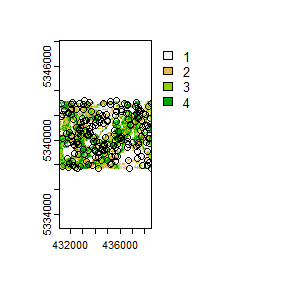#> Simple feature collection with 200 features and 1 field
#> Geometry type: POINT
#> Dimension:     XY
#> Bounding box:  xmin: 431290 ymin: 5337710 xmax: 438530 ymax: 5343230
#> Projected CRS: UTM Zone 17, Northern Hemisphere
#> First 10 features:
#>    strata               geometry
#> 1       1 POINT (436570 5340230)
#> 2       1 POINT (436570 5340230)
#> 3       1 POINT (438210 5338330)
#> 4       1 POINT (435290 5338710)
#> 5       1 POINT (434930 5342110)
#> 6       1 POINT (433510 5340970)
#> 7       1 POINT (434370 5342170)
#> 8       1 POINT (435770 5338710)
#> 9       1 POINT (435750 5342350)
#> 10      1 POINT (434610 5342010)

## sample_nc

sample_nc() function implements the Nearest Centroid sampling algorithm described in Melville & Stone (2016). The algorithm uses kmeans clustering where the number of clusters (centroids) is equal to the desired sample size (nSamp).

Cluster centers are located, which then prompts the nearest neighbour mraster pixel for each cluster to be selected (assuming default k parameter). These nearest neighbours are the output sample units.

#--- perform simple random sampling ---#
sample_nc(mraster = mraster, # input
nSamp = 25, # desired sample size
plot = TRUE)
#> K-means being performed on 3 layers with 25 centers.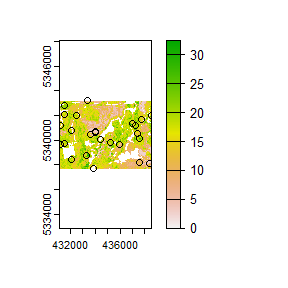#> Simple feature collection with 25 features and 4 fields
#> Geometry type: POINT
#> Dimension:     XY
#> Bounding box:  xmin: 431150 ymin: 5337710 xmax: 438510 ymax: 5343190
#> CRS:           +proj=utm +zone=17 +ellps=GRS80 +units=m +no_defs
#> First 10 features:
#>        zq90 pzabove2  zsd kcenter               geometry
#> 50298 15.60     48.9 4.29       1 POINT (437410 5340550)
#> 23567 18.50     64.2 5.51       2 POINT (432450 5341970)
#> 859    6.19     60.5 1.45       3 POINT (433350 5343190)
#> 83289 23.80     89.8 6.83       4 POINT (433290 5338770)
#> 67385  8.54     40.4 2.25       5 POINT (435990 5339630)
#> 37306 16.80     88.1 4.19       6 POINT (431210 5341230)
#> 63615 19.50     20.9 6.20       7 POINT (435190 5339830)
#> 49010  4.55     30.7 1.02       8 POINT (434030 5340610)
#> 37981 19.80     92.2 4.25       9 POINT (437250 5341210)
#> 23870 14.60     88.4 3.51      10 POINT (438510 5341970)

Altering the k parameter leads to a multiplicative increase in output sample units where total output samples = $$nSamp * k$$.

#--- perform simple random sampling ---#
samples <- sample_nc(mraster = mraster, # input
k = 2, # number of nearest neighbours to take for each kmeans center
nSamp = 25, # desired sample size
plot = TRUE)
#> K-means being performed on 3 layers with 25 centers.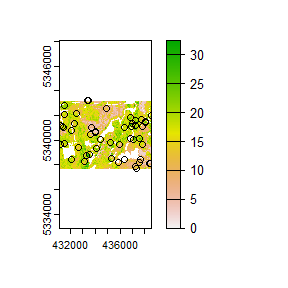#--- total samples = nSamp * k (25 * 2) = 50 ---#
nrow(samples)
#>  50

Visualizing what the kmeans centers and sample units looks like is possible when using details = TRUE. The $kplot output provides a quick visualization of where the centers are based on a scatter plot of the first 2 layers in mraster. Notice that the centers are well distributed in covariate space and chosen sample units are the closest pixels to each center (nearest neighbours). #--- perform simple random sampling with details ---# details <- sample_nc(mraster = mraster, # input nSamp = 25, # desired sample number details = TRUE) #> K-means being performed on 3 layers with 25 centers. #--- plot ggplot output ---# details$kplot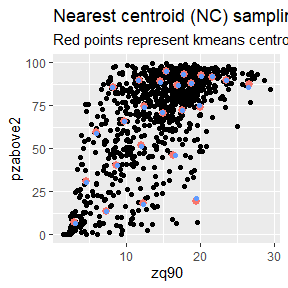## sample_clhs

sample_clhs() function implements conditioned Latin hypercube (clhs) sampling methodology from the clhs package.

TIP!

A number of other functions in the sgsR package help to provide guidance on clhs sampling including calculate_pop() and calculate_lhsOpt(). Check out these functions to better understand how sample numbers could be optimized.

The syntax for this function is similar to others shown above, although parameters like iter, which define the number of iterations within the Metropolis-Hastings process are important to consider. In these examples we use a low iter value for efficiency. Default values for iter within the clhs package are 10,000.

sample_clhs(mraster = mraster, # input
nSamp = 200, # desired sample size
plot = TRUE, # plot
iter = 100) # number of iterations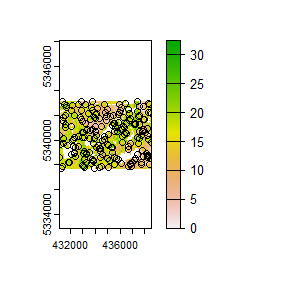The cost parameter defines the mraster covariate, which is used to constrain the clhs sampling. An example could be the distance a pixel is from road access (e.g. from calculate_distance() see example below), terrain slope, the output from calculate_coobs(), or many others.

#--- cost constrained examples ---#
#--- calculate distance to access layer for each pixel in mr ---#
mr.c <- calculate_distance(raster = mraster, # input
access = access, # define access road network
plot = TRUE) # plot
#>
|---------|---------|---------|---------|
=========================================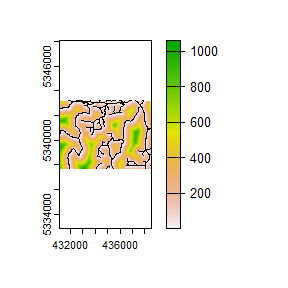sample_clhs(mraster = mr.c, # input
nSamp = 250, # desired sample size
iter = 100, # number of iterations
cost = "dist2access", # cost parameter - name defined in calculate_distance()
plot = TRUE) # plot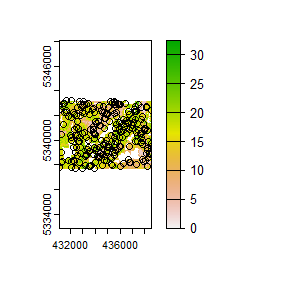## sample_balanced

The sample_balanced() algorithm performs a balanced sampling methodology from the stratifyR / SamplingBigData packages.

sample_balanced(mraster = mraster, # input
nSamp = 200, # desired sample size
plot = TRUE) # plot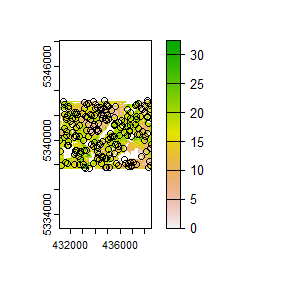#> Simple feature collection with 200 features and 0 fields
#> Geometry type: POINT
#> Dimension:     XY
#> Bounding box:  xmin: 431110 ymin: 5337730 xmax: 438530 ymax: 5343210
#> CRS:           +proj=utm +zone=17 +ellps=GRS80 +units=m +no_defs
#> First 10 features:
#>                  geometry
#> 1  POINT (434770 5343210)
#> 2  POINT (438210 5343210)
#> 3  POINT (433830 5343170)
#> 4  POINT (431390 5343150)
#> 5  POINT (433090 5343090)
#> 6  POINT (438250 5343090)
#> 7  POINT (433430 5343010)
#> 8  POINT (437530 5342970)
#> 9  POINT (434890 5342910)
#> 10 POINT (437350 5342910)
sample_balanced(mraster = mraster, # input
nSamp = 100, # desired sample size
algorithm = "lcube", # algorithm type
access = access, # define access road network
buff_inner = 50, # inner buffer - no sample units within this distance from road
buff_outer = 200) # outer buffer - no sample units further than this distance from road
#> Simple feature collection with 100 features and 0 fields
#> Geometry type: POINT
#> Dimension:     XY
#> Bounding box:  xmin: 431590 ymin: 5337710 xmax: 438430 ymax: 5343230
#> CRS:           +proj=utm +zone=17 +ellps=GRS80 +units=m +no_defs
#> First 10 features:
#>                  geometry
#> 1  POINT (433970 5342890)
#> 2  POINT (433810 5341270)
#> 3  POINT (434070 5339170)
#> 4  POINT (434990 5343130)
#> 5  POINT (433750 5342650)
#> 6  POINT (433030 5342050)
#> 7  POINT (435110 5339790)
#> 8  POINT (437930 5343110)
#> 9  POINT (433730 5340630)
#> 10 POINT (434870 5337970)

## sample_ahels

The sample_ahels() function performs the adapted Hypercube Evaluation of a Legacy Sample (ahels) algorithm usingexisting sample data and an mraster. New sample units are allocated based on quantile ratios between the existing sample and mraster covariate dataset.

This algorithm was adapted from that presented in the paper below, which we highly recommend.

Malone BP, Minansy B, Brungard C. 2019. Some methods to improve the utility of conditioned Latin hypercube sampling. PeerJ 7:e6451 DOI 10.7717/peerj.6451

This algorithm:

1. Determines the quantile distributions of existing sample units and mraster covariates.

2. Determines quantiles where there is a disparity between sample units and covariates.

3. Prioritizes sampling within those quantile to improve representation.

To use this function, user must first specify the number of quantiles (nQuant) followed by either the nSamp (total number of desired sample units to be added) or the threshold (sampling ratio vs. covariate coverage ratio for quantiles - default is 0.9) parameters.

#--- remove type variable from existing  - causes plotting issues ---#

existing <- existing %>% select(-type)

sample_ahels(mraster = mraster,
existing = existing, # existing sample
plot = TRUE) # plot
#> Simple feature collection with 265 features and 7 fields
#> Geometry type: POINT
#> Dimension:     XY
#> Bounding box:  xmin: 431110 ymin: 5337710 xmax: 438530 ymax: 5343230
#> CRS:           +proj=utm +zone=17 +ellps=GRS80 +units=m +no_defs
#> First 10 features:
#>      type.x  zq90 pzabove2  zsd strata type.y  rule               geometry
#> 1  existing  8.84     57.4 2.24      1    new rule1 POINT (435970 5339490)
#> 2  existing  7.33     66.6 1.73      1    new rule2 POINT (436470 5339550)
#> 3  existing  5.43     78.2 1.21      1    new rule2 POINT (435550 5342350)
#> 4  existing  4.57     68.3 1.01      1    new rule2 POINT (433810 5341570)
#> 5  existing 10.80     70.0 2.82      1    new rule2 POINT (436950 5338530)
#> 6  existing  9.15     97.6 1.92      1    new rule2 POINT (436670 5338470)
#> 7  existing  9.74     36.1 2.59      1    new rule2 POINT (436750 5339070)
#> 8  existing  8.98     53.3 2.38      1    new rule2 POINT (436010 5341590)
#> 9  existing 10.10     78.0 2.40      1    new rule2 POINT (431970 5341490)
#> 10 existing  3.73     40.7 0.72      1    new rule2 POINT (434350 5340890)

TIP!

Notice that no threshold, nSamp, or nQuant were defined. That is because the default setting for threshold = 0.9 and nQuant = 10.

The first matrix output shows the quantile ratios between the sample and the covariates. A value of 1.0 indicates that the sample is representative of quantile coverage. Values > 1.0 indicate over representation of sample units, while < 1.0 indicate under representation.

sample_ahels(mraster = mraster,
existing = existing, # existing sample
nQuant = 20, # define 20 quantiles
nSamp = 300) # desired sample size
#> Simple feature collection with 500 features and 7 fields
#> Geometry type: POINT
#> Dimension:     XY
#> Bounding box:  xmin: 431110 ymin: 5337710 xmax: 438530 ymax: 5343230
#> CRS:           +proj=utm +zone=17 +ellps=GRS80 +units=m +no_defs
#> First 10 features:
#>      type.x  zq90 pzabove2  zsd strata type.y  rule               geometry
#> 1  existing  8.84     57.4 2.24      1    new rule1 POINT (435970 5339490)
#> 2  existing  7.33     66.6 1.73      1    new rule2 POINT (436470 5339550)
#> 3  existing  5.43     78.2 1.21      1    new rule2 POINT (435550 5342350)
#> 4  existing  4.57     68.3 1.01      1    new rule2 POINT (433810 5341570)
#> 5  existing 10.80     70.0 2.82      1    new rule2 POINT (436950 5338530)
#> 6  existing  9.15     97.6 1.92      1    new rule2 POINT (436670 5338470)
#> 7  existing  9.74     36.1 2.59      1    new rule2 POINT (436750 5339070)
#> 8  existing  8.98     53.3 2.38      1    new rule2 POINT (436010 5341590)
#> 9  existing 10.10     78.0 2.40      1    new rule2 POINT (431970 5341490)
#> 10 existing  3.73     40.7 0.72      1    new rule2 POINT (434350 5340890)

Notice that the total number of samples is 500. This value is the sum of existing units (200) and number of sample units defined by nSamp = 300.

## sample_existing

Acknowledging that existing sample networks exist is important. There is significant investment into these samples, and in order to keep inventories up-to-date, we often need to collect new data at these locations. The sample_existing algorithm provides a method for sub-sampling an existing sample network should the financial / logistical resources not be available to collect data at all sample units. The algorithm leverages latin hypercube sampling using the clhs package to effectively sample within an existing network.

The algorithm has two fundamental approaches:

1. Sample exclusively using the sample network and the attributes it contains.

2. Should raster information be available and co-located with the sample, use these data as population values to improve sub-sampling of existing.

Much like the sample_clhs() algorithm, users can define a cost parameter, which will be used to constrain sub-sampling. A cost parameter is a user defined metric/attribute such as distance from roads (e.g. calculate_distance()), elevation, etc.

### Basic sub-sampling of existing

First we can create an existing sample for our example. Lets imagine we have a dataset of ~900 samples, and we know we only have resources to sample 300 of them. We have some ALS data available (mraster), which we will use as our distributions to sample within.

#--- generate existing samples and extract metrics ---#
existing <- sample_srs(raster = mraster, nSamp = 900, plot = TRUE)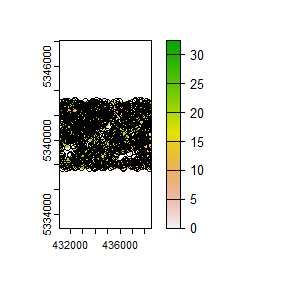Now lets sub-sample.

#--- sub sample using ---#
e <- existing %>%
extract_metrics(mraster = mraster, existing = .)

sample_existing(existing = e, nSamp = 300, plot = TRUE)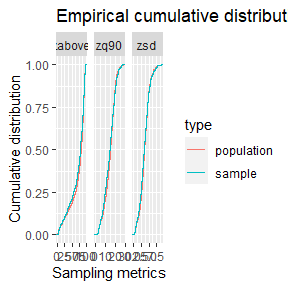#> Simple feature collection with 300 features and 3 fields
#> Geometry type: POINT
#> Dimension:     XY
#> Bounding box:  xmin: 431110 ymin: 5337710 xmax: 438550 ymax: 5343230
#> CRS:           +proj=utm +zone=17 +ellps=GRS80 +units=m +no_defs
#> First 10 features:
#>      zq90 pzabove2  zsd               geometry
#> 351  6.20      2.8 1.50 POINT (433870 5342730)
#> 137 21.60     86.8 6.08 POINT (435630 5341690)
#> 299 17.30     91.3 3.66 POINT (433150 5339130)
#> 302 16.40     84.7 4.20 POINT (435290 5340210)
#> 663  9.58     60.4 2.55 POINT (438170 5338250)
#> 112 11.00     98.0 2.51 POINT (436930 5337870)
#> 233 19.60     87.0 5.46 POINT (431210 5342810)
#> 611 27.70     74.8 8.54 POINT (436830 5341330)
#> 81  20.20     51.5 7.33 POINT (435430 5340170)
#> 517 19.70     91.4 6.29 POINT (431230 5339350)

TIP!

Notice that we used extract_metrics() after creating our existing. If the user provides a raster for the algorithm this isn’t neccesary (its done internally). If only sample units are given, attributes must be provided and sampling will be conducted on all included attributes.

We see from the output that we get 300 sample units that are a sub-sample of existing. The plotted output shows cumulative frequency distributions of the population (all existing samples) and the sub-sample (the 300 samples we requested).

### Sub-sampling using raster distributions

Our systematic sample of ~900 plots is fairly comprehensive, however we can generate a true population distribution through the inclusion of the ALS metrics in the sampling process. The metrics will be included in internal latin hypercube sampling to help guide sub-sampling of existing.

#--- sub sample using ---#
sample_existing(existing = existing, # our existing sample
nSamp = 300, # desired sample size
raster = mraster, # include mraster metrics to guide sampling of existing
plot = TRUE) # plot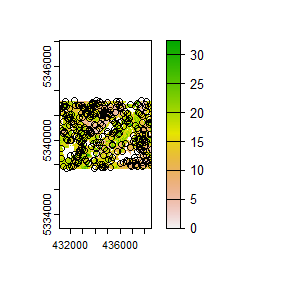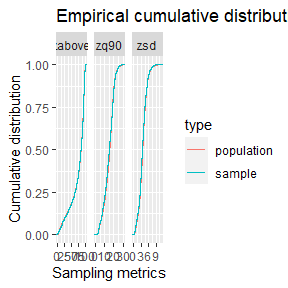#> Simple feature collection with 300 features and 3 fields
#> Geometry type: POINT
#> Dimension:     XY
#> Bounding box:  xmin: 431170 ymin: 5337710 xmax: 438550 ymax: 5343230
#> CRS:           +proj=utm +zone=17 +ellps=GRS80 +units=m +no_defs
#> First 10 features:
#>      zq90 pzabove2  zsd               geometry
#> 475 15.30     85.9 3.88 POINT (436470 5342310)
#> 133 10.70     74.2 3.03 POINT (437290 5338350)
#> 592 20.20     81.6 5.19 POINT (431690 5342690)
#> 84  21.10     86.2 6.11 POINT (436150 5341510)
#> 66  22.20     89.2 5.92 POINT (438550 5341390)
#> 760  3.96      9.5 0.88 POINT (437570 5339210)
#> 362 19.40     90.9 4.92 POINT (433250 5338250)
#> 327  4.08     32.2 0.80 POINT (433610 5340730)
#> 253 23.00     88.4 6.96 POINT (431490 5342750)
#> 65   7.75     76.2 1.70 POINT (436750 5338290)

The sample distribution again mimics the population distribution quite well! Now lets try using a cost variable to constrain the sub-sample.

#--- create distance from roads metric ---#
dist <- calculate_distance(raster = mraster, access = access)
#>
|---------|---------|---------|---------|
=========================================

#--- sub sample using ---#
sample_existing(existing = existing, # our existing sample
nSamp = 300, # desired sample size
raster = dist, # include mraster metrics to guide sampling of existing
cost = 4, # either provide the index (band number) or the name of the cost layer
plot = TRUE) # plot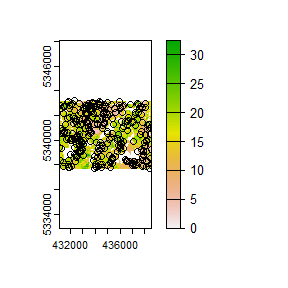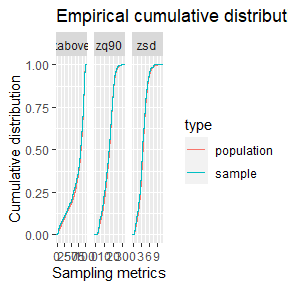#> Simple feature collection with 300 features and 4 fields
#> Geometry type: POINT
#> Dimension:     XY
#> Bounding box:  xmin: 431130 ymin: 5337750 xmax: 438550 ymax: 5343230
#> CRS:           +proj=utm +zone=17 +ellps=GRS80 +units=m +no_defs
#> First 10 features:
#>      zq90 pzabove2  zsd dist2access               geometry
#> 281 12.80     85.3 2.48   105.49139 POINT (431890 5342590)
#> 554  9.94     47.8 2.81   175.00446 POINT (434870 5341810)
#> 845 19.20     79.9 4.88   155.44493 POINT (434350 5339610)
#> 702  9.54     97.3 1.86    44.23426 POINT (437270 5337950)
#> 784 20.40     95.6 3.39   445.63487 POINT (437230 5341230)
#> 444 19.90     86.6 4.41   770.02241 POINT (435470 5341290)
#> 516 12.70     71.1 3.37    39.69336 POINT (434850 5342690)
#> 84  21.10     86.2 6.11   235.00692 POINT (436150 5341510)
#> 537 21.30     84.4 6.51    65.41171 POINT (437250 5342110)
#> 706 17.00     81.6 3.74   150.58222 POINT (432510 5341650)

Finally, should the user wish to further constrain the sample based on access like other sampling approaches in sgsR that is also possible.

#--- ensure access and existing are in the same CRS ---#

sf::st_crs(existing) <- sf::st_crs(access)

#--- sub sample using ---#
sample_existing(existing = existing, # our existing sample
nSamp = 300, # desired sample size
raster = dist, # include mraster metrics to guide sampling of existing
cost = 4, # either provide the index (band number) or the name of the cost layer
access = access, # roads layer
buff_inner = 50, # inner buffer - no sample units within this distance from road
buff_outer = 300, # outer buffer - no sample units further than this distance from road
plot = TRUE) # plot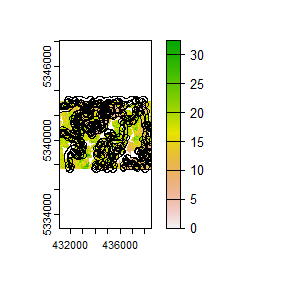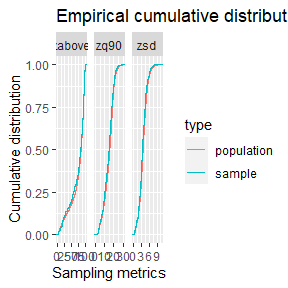#> Simple feature collection with 300 features and 4 fields
#> Geometry type: POINT
#> Dimension:     XY
#> Bounding box:  xmin: 431190 ymin: 5337750 xmax: 438530 ymax: 5343230
#> Projected CRS: UTM_Zone_17_Northern_Hemisphere
#> First 10 features:
#>      zq90 pzabove2  zsd dist2access               geometry
#> 408  4.77     23.9 1.08   178.60875 POINT (433470 5341190)
#> 309  7.29     32.5 1.86   115.23022 POINT (433650 5340590)
#> 196 20.00     72.1 6.53    76.21352 POINT (438510 5338910)
#> 466 14.80     97.6 3.12   163.61179 POINT (433910 5337750)
#> 188 28.60     93.6 5.50   216.21364 POINT (432350 5338810)
#> 397 27.80     93.1 6.96   199.60984 POINT (436730 5341370)
#> 478  4.47     17.9 1.55    80.52950 POINT (434150 5341310)
#> 241 18.70     95.3 3.96   184.36887 POINT (435530 5337930)
#> 230 17.00     72.8 4.25   238.64183 POINT (437850 5340070)
#> 313 14.90     79.6 3.34   124.56134 POINT (432990 5342770)

TIP!

The greater constraints we add to sampling, the less likely we will have strong correlations between the population and sample, so its always important to understand these limitations and plan accordingly.